# 1.学习总结(2分)

## 1.1图的思维导图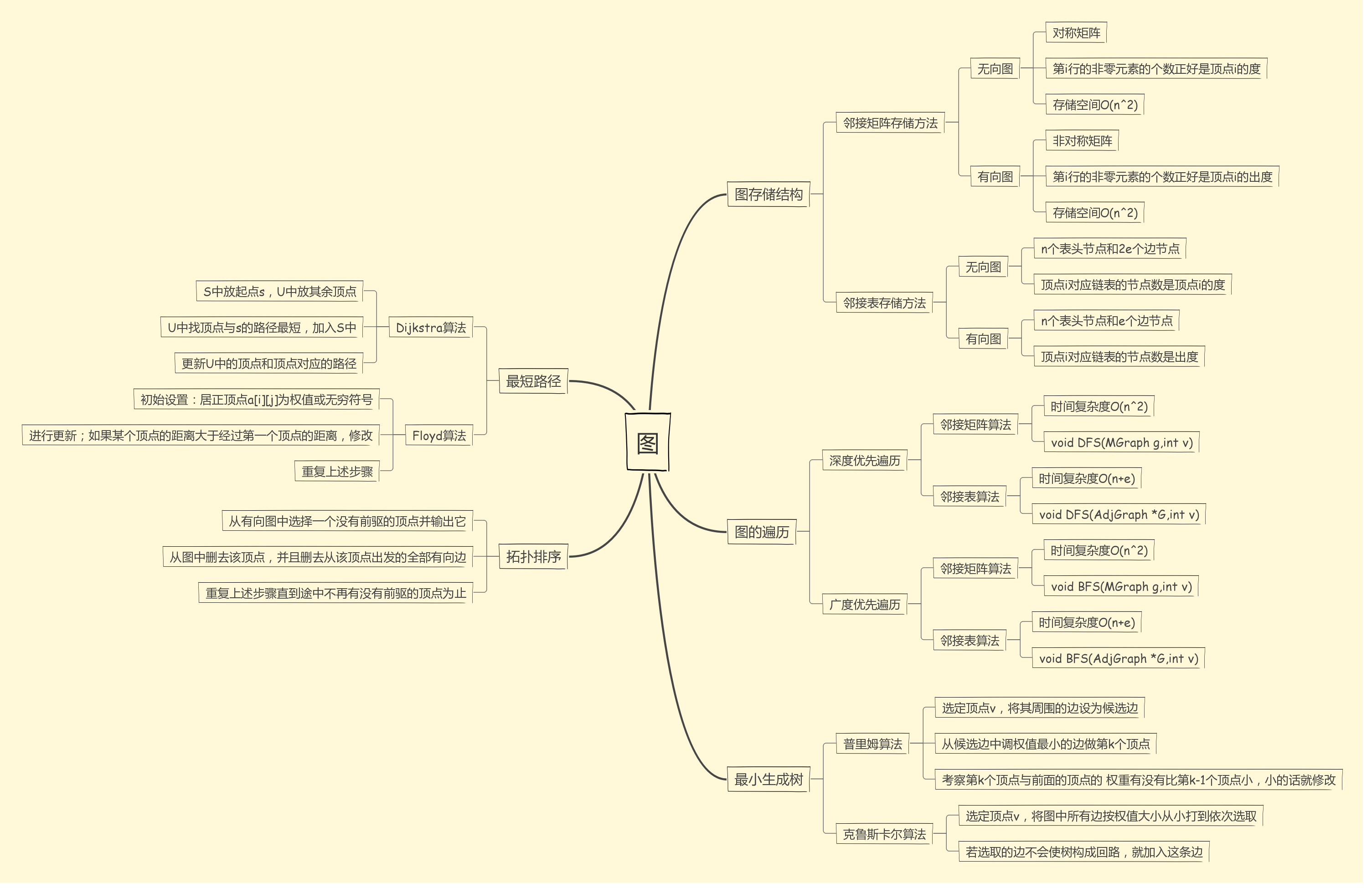# 2.PTA实验作业（4分）

## 2.2 设计思路（伪代码或流程图）

1.构建图结构体，定义全局变量visited[]用来标记是否遍历
2.建邻接矩阵的图，输入它们之间的关系（-1    0    1  三种情况）；
3.for（i=0        to          count）{
输入顶点k1，k2；
如果关系为1，是朋友；
如果关系为0，不是朋友也不是敌人；
如果关系为-1，{
广度遍历有没有共同朋友；
有的话输出“OK，  but。。。”
没有的话是敌人；
}
4.return 0；


## 2.3 代码截图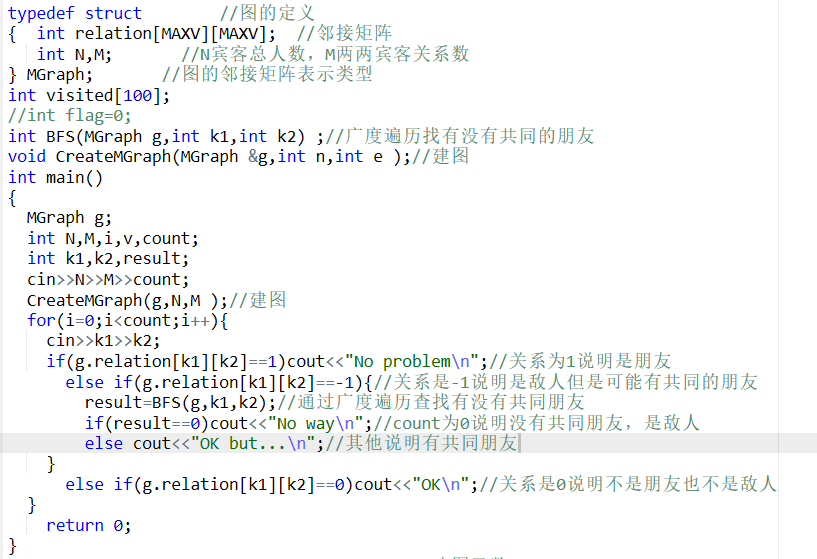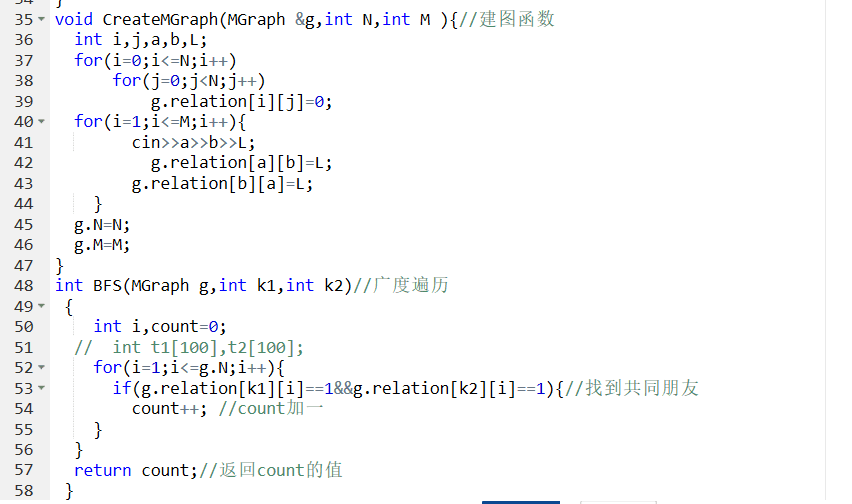## 2.4 PTA提交列表说明。## 2.2 设计思路（伪代码或流程图）

1.定义各个全局变量，如代码截图；其中visited[]数组记录所有顶点是否都被遍历；
2.输入顶点数n，和边树e；
3.入宫边数小于顶点数，输出-1；
4.建邻接矩阵图，填入权值；
5.普里姆函数找最小生成树；
6.如果visited[]数组中有未被遍历的点，输出-1；否则输出flag，即最低成本；
7.普里姆函数     prim(int v){
定义lowcost[]和closest[]数组以及min存放实时最小成本；
给两个数组赋初值   lowcost[i]=edges[v][i];closest[i]=v;
for   (i=0          to        n){
min定初值无穷大；
for(j=1  to    n)如果lowcost数组不为0且小于min，min值更新，k=j；
最低成本flag=flag+1；
两个数组重新赋值0和1；
修改数组的值           lowcost[j]=edges[k][j];closest[j]=k;
}


## 2.3 代码截图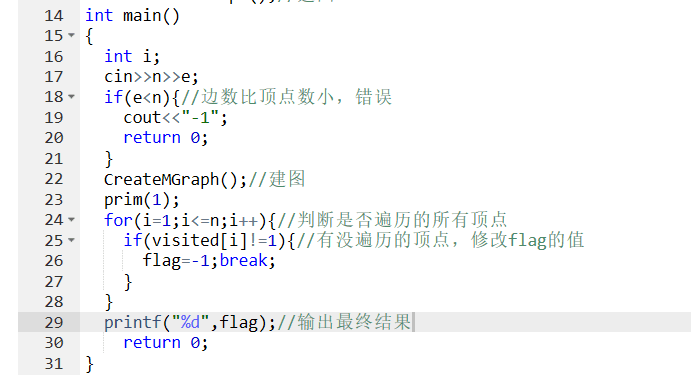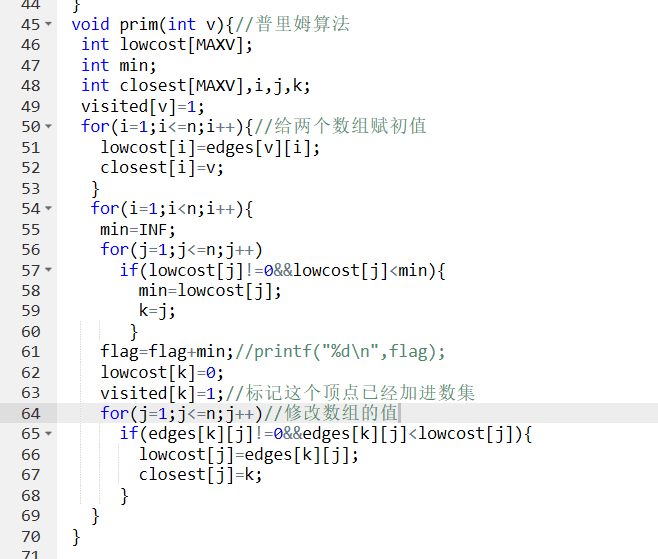## 2.4 PTA提交列表说明。## 2.2 设计思路

1。定义各个全局变量数组，模仿邻接矩阵的数组形式
2.给路径数组和开销数组赋初值为501；
3.根据输入修改路径数组和开销数组的值；
4.用两个新的数组记录S出发城市下的到每个城市的路径长度和开销；
5.Dijkstra算法求最小路径长度和开销；
6.输出路径长度和开销；


## 2.3 代码截图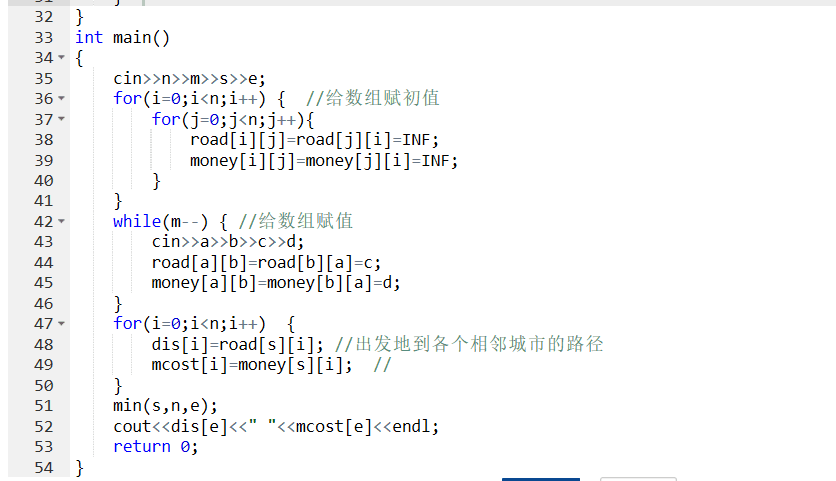## 2.4 PTA提交列表说明。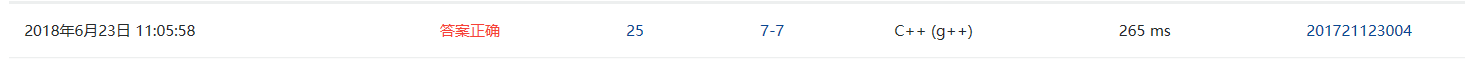# 3.截图本周题目集的PTA最后排名（3分）

## 3.1 PTA排名2分

# 4. 阅读代码（必做，1分）

#include <stdio.h>
#include<stdlib.h>
#define FULL 250001
typedef struct node *Node;
typedef struct {
int Node;//目标结点
int Lenth;//边长
} data;
struct node {
data Reached;//与之有边连接的结点集合
int right;//集合里的结点个数
};

int teams;//保存每个结点带有的救援队数目
int Lenth;//记录从起点出发到各结点的路径长度 ;记录该路径能获取的救援队总数；【2】记录等长路径条数
Node Map;//地图

int cmp(const void*a,const void*b) {//
data*x=(data*)a;
data*y=(data*)b;
if(x->Lenth!=y->Lenth) {
return x->Lenth-y->Lenth;
} else if(teams[x->Node]!=teams[y->Node]) {
return teams[y->Node]-teams[x->Node];
} else  return teams[x->Node]-teams[y->Node];
}

void Dijkstra(int);
void DFS(int,const int);
int main() {
int N,M,S,D;//结点数、边数、起点、终点
scanf("%d%d%d%d",&N,&M,&S,&D);

Map=(Node)malloc(sizeof(struct node)*N);//初始化地图上的结点
for(int i=0; i<N; Map[i++].right=0);

for(int i=0; i<N; scanf("%d",&teams[i++]));//读入每个结点的价值——城市拥有的救援队数量

{
//读入地图信息，并排序整理
while(M--) {
int a,b,lenth;
scanf("%d%d%d",&a,&b,&lenth);
Map[a].Reached[Map[a].right].Node=b;
Map[a].Reached[Map[a].right++].Lenth=lenth;
Map[b].Reached[Map[b].right].Node=a;
Map[b].Reached[Map[b].right++].Lenth =lenth;
}
for(int i=0; i<N; i++) {
//          printf("\n%d:",i);
qsort(Map[i].Reached,Map[i].right,sizeof(data),cmp);
for(int j=0; j<Map[i].right; j++) {
//              printf("{%d-%d}",Map[i].Reached[j].Node,Map[i].Reached[j].Lenth);
}
}
}

for(int i=0; i<N; Lenth[i++]=FULL); //初始化设定从起点到所有其他结点的路径长度为无穷;
Lenth[S]=0;
Lenth[S]=teams[S];
Dijkstra(S);
printf("%d %d\n",Lenth[D],Lenth[D]);
DFS(D,S);
printf("%d",D);
return 0;
}

void Dijkstra(int a) {//戴克斯特拉算法

for(int i=0; i<Map[a].right; i++) {
data temp=Map[a].Reached[i];
if(Lenth[a]+temp.Lenth<Lenth[temp.Node]) {
Lenth[temp.Node]=Lenth[a]+temp.Lenth;
Lenth[temp.Node]=Lenth[a]+teams[temp.Node];
Lenth[temp.Node]=1;
Dijkstra(temp.Node);
} else if(Lenth[a]+temp.Lenth==Lenth[temp.Node]) {
if(Lenth[a]+teams[temp.Node]>Lenth[temp.Node]) {
Lenth[temp.Node]=Lenth[a]+teams[temp.Node];
}
++Lenth[temp.Node];
Dijkstra(temp.Node);
}
}

}

void DFS(int dd,const int S) {//深度优先，从终点递归回溯， 如果存在某个结点：它到终点的边长加上它到起点的长度== 终点到原点的长度&&它到起点的救援队数量+终点的救援队数量== 起点到终点的救援队数量，那么这个结点一定是最短路上的一个结点。
if(dd==S)return;
for(int i=0; i<Map[dd].right; i++) {
data temp=Map[dd].Reached[i];
if(Lenth[temp.Node]+temp.Lenth==Lenth[dd]&&
Lenth[temp.Node]+teams[dd]==Lenth[dd]) {
//          printf("*");
DFS(temp.Node,S);
printf("%d ",temp.Node);
}
}
}


# 5.码云提交记录

posted @ 2018-06-17 21:58  一叶落而秋将至  阅读(318)  评论(0编辑  收藏  举报